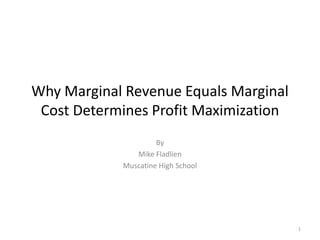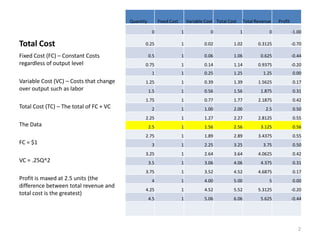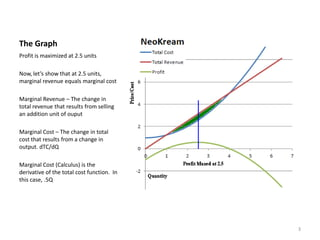Successfully reported this slideshow.×
1 of 8

Why marginal revenue equals marginal cost determines profit

6

Share

This Slideware explains to students in an AP Microeconomics class why marginal revenue equals marginal cost maximizes profit. Students seldom make this connection and just memorize the formula. This presentation makes teaching the concept easy.

See all

See all

Why marginal revenue equals marginal cost determines profit

1. 1. Why Marginal Revenue Equals Marginal Cost Determines Profit Maximization By Mike Fladlien Muscatine High School 1
2. 2. Quantity Fixed Cost Variable Cost Total Cost Total Revenue Profit 0 1 0 1 0 -1.00 Total Cost 0.25 1 0.02 1.02 0.3125 -0.70 Fixed Cost (FC) – Constant Costs 0.5 1 0.06 1.06 0.625 -0.44 regardless of output level 0.75 1 0.14 1.14 0.9375 -0.20 1 1 0.25 1.25 1.25 0.00 Variable Cost (VC) – Costs that change 1.25 1 0.39 1.39 1.5625 0.17 over output such as labor 1.5 1 0.56 1.56 1.875 0.31 1.75 1 0.77 1.77 2.1875 0.42 Total Cost (TC) – The total of FC + VC 2 1 1.00 2.00 2.5 0.50 2.25 1 1.27 2.27 2.8125 0.55 The Data 2.5 1 1.56 2.56 3.125 0.56 2.75 1 1.89 2.89 3.4375 0.55 FC = \$1 3 1 2.25 3.25 3.75 0.50 3.25 1 2.64 3.64 4.0625 0.42 VC = .25Q^2 3.5 1 3.06 4.06 4.375 0.31 3.75 1 3.52 4.52 4.6875 0.17 Profit is maxed at 2.5 units (the 4 1 4.00 5.00 5 0.00 difference between total revenue and 4.25 1 4.52 5.52 5.3125 -0.20 total cost is the greatest) 4.5 1 5.06 6.06 5.625 -0.44 2
3. 3. The Graph Profit is maximized at 2.5 units Now, let’s show that at 2.5 units, marginal revenue equals marginal cost Marginal Revenue – The change in total revenue that results from selling an addition unit of ouput Marginal Cost – The change in total cost that results from a change in output. dTC/dQ Marginal Cost (Calculus) is the derivative of the total cost function. In this case, .5Q 3
4. 4. MR = MC The slope of the Total Revenue, TR, curve is the Marginal Revenue, MR. The Slope of the Total Cost, TC, curve is the Marginal Cost curve. Let’s look at the slope of the Total Revenue, TR, curve. The price of the product is \$1.25 Perfectly Competitive so the firm can sell all they want at \$1.25 Price Taker selling a homogenous good with many buyers and sellers 4
5. 5. MR = MC Slope of TC is Marginal Cost At \$1.25 the quantity is 2.5 5
6. 6. MR = MC The intersection of the Marginal Revenue and Marginal Cost curves is the profit maximization point. Why? Because the distance between total revenue and total cost is the greatest at that point. 6
7. 7. But Why Does This Work? When I show this to students, they still don’t get it. To convince your students that MR = MC maximizes profits, you have to show that the slopes are equal. At 2.5 units the distance between the two curves is the farthest so profit is maximized. 7
8. 8. The End www.mikeroeconomics.blogspot.com 8